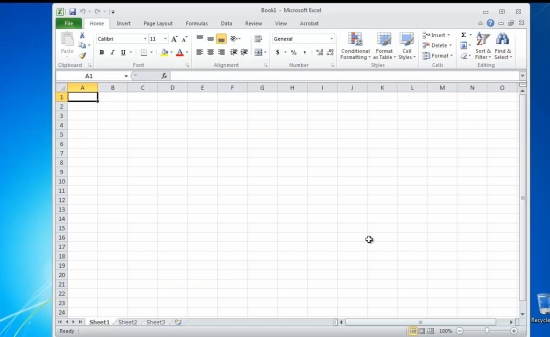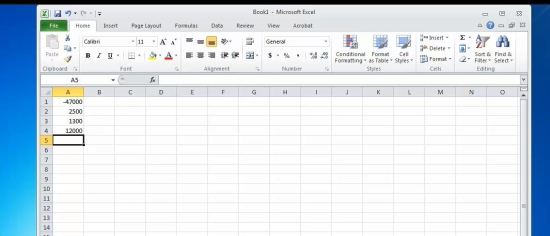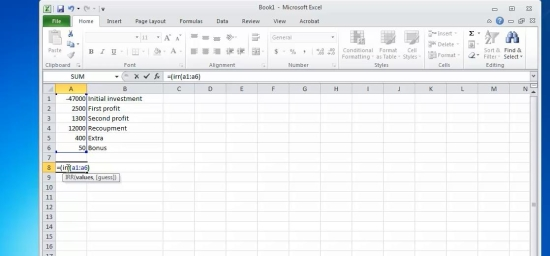## How to Calculate the Internal Rate of Return, or IRR, in Microsoft Excel

In this tutorial you will learn how to calculate the internal rate of return, or IRR, in Microsoft Excel.

The internal rate of return is a financial statistical function that calculates the interest rate one receives for an investment consisting of payments and income that appear at regular intervals.

This simple function will be enough to display if and when your investment pays back over time.

Here’s how to calculate it.

Prerequisites: Microsoft Word.

Step 1. Launch Excel and open up the document containing the projected or calculated amounts.Step 2. Arrange them under one another, as they are given to you by the bank and by your production department.Step 3. Position the cursor in the cell you want to put your result to.

Type the following formula:=IRR (AAxx:AAyy),

Where AAxx and AAyy are the start and the end of your column. Note that debts will always be represented with negative value, wheres income will be considered positive.

Press Enter. Excel is calculating your result.Result: Congratulations, you have learnt how to calculate the internal rate of return.